JMSLTM Numerical Library 7.2.0
com.imsl.stat

## Class ChiSquaredTest

• ```public class ChiSquaredTest
extends Object```
Chi-squared goodness-of-fit test.

`ChiSquaredTest` performs a chi-squared goodness-of-fit test that a random sample of observations is distributed according to a specified theoretical cumulative distribution. The theoretical distribution, which may be continuous, discrete, or a mixture of discrete and continuous distributions, is specified via a user-defined function F where F implements `CdfFuntion`. Because the user is allowed to specify a range for the observations in the `setRange` method, a test that is conditional upon the specified range is performed.

`ChiSquaredTest` can be constructed in two different ways. The intervals can be specified via the array cutpoints. Otherwise, the number of cutpoints can be given and equiprobable intervals computed by the constructor. The observations are divided into these intervals. Regardless of the method used to obtain them, the intervals are such that the lower endpoint is not included in the interval while the upper endpoint is always included. The user should determine the cutpoints when the cumulative distribution function has discrete elements since `ChiSquaredTest` cannot determine them in this case.

By default, the lower and upper endpoints of the first and last intervals areand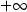, respectively. The method `setRange` can be used to change the range.

A tally of counts is maintained for the observations in x as follows:

If the cutpoints are specified by the user, the tally is made in the interval to which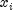belongs, using the user-specified endpoints.

If the cutpoints are determined by the class then the cumulative probability at,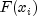, is computed using `cdf`.

The tally foris made in interval number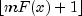, where m is the number of categories and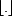is the function that takes the greatest integer that is no larger than the argument of the function. If the cutpoints are specified by the user, the tally is made in the interval to whichbelongs using the endpoints specified by the user. Thus, if the computer time required to calculate the cumulative distribution function is large, user-specified cutpoints may be preferred in order to reduce the total computing time.

If the expected count in any cell is less than 1, then a rule of thumb is that the chi-squared approximation may be suspect. A warning message to this effect is issued in this case, as well as when an expected value is less than 5.

Example
• ### Nested Class Summary

Nested Classes
Modifier and Type Class and Description
`static class ` `ChiSquaredTest.DidNotConvergeException`
The iteration did not converge
`static class ` `ChiSquaredTest.NoObservationsException`
There are no observations.
`static class ` `ChiSquaredTest.NotCDFException`
The function is not a Cumulative Distribution Function (CDF).
• ### Constructor Summary

Constructors
Constructor and Description
```ChiSquaredTest(CdfFunction cdf, double[] cutpoints, int nParameters)```
Constructor for the Chi-squared goodness-of-fit test.
```ChiSquaredTest(CdfFunction cdf, int nCutpoints, int nParameters)```
Constructor for the Chi-squared goodness-of-fit test
• ### Method Summary

Methods
Modifier and Type Method and Description
`double[]` `getCellCounts()`
Returns the cell counts.
`double` `getChiSquared()`
Returns the chi-squared statistic.
`double[]` `getCutpoints()`
Returns the cutpoints.
`double` `getDegreesOfFreedom()`
Returns the degrees of freedom in chi-squared.
`double[]` `getExpectedCounts()`
Returns the expected counts.
`double` `getP()`
Returns the p-value for the chi-squared statistic.
`void` `setCutpoints(double[] cutpoints)`
Sets the cutpoints.
`void` ```setRange(double lower, double upper)```
Sets endpoints of the range of the distribution.
`void` `update(double x)`
Adds a new observation to the test.
`void` `update(double[] x)`
Adds new observations to the test.
`void` ```update(double[] x, double[] freq)```
Adds new observations to the test.
`void` ```update(double x, double freq)```
Adds a new observation to the test.
• ### Methods inherited from class java.lang.Object

`clone, equals, finalize, getClass, hashCode, notify, notifyAll, toString, wait, wait, wait`
• ### Constructor Detail

• #### ChiSquaredTest

```public ChiSquaredTest(CdfFunction cdf,
double[] cutpoints,
int nParameters)
throws ChiSquaredTest.NotCDFException```
Constructor for the Chi-squared goodness-of-fit test.
Parameters:
`cdf` - a `CdfFunction` object that implements the CdfFunction interface
`cutpoints` - a `double` array containing the cutpoints
`nParameters` - an `int` which specifies the number of parameters estimated in computing the Cdf. For example, with a binomial distribution `nParameters`=1 if p is estimated from the data and `nParameters`=0 if p is given in advance. The degrees of freedom in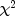is: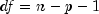where n = number or non-empty cells and p = `nParameters`.
Throws:
`ChiSquaredTest.NotCDFException`
• #### ChiSquaredTest

```public ChiSquaredTest(CdfFunction cdf,
int nCutpoints,
int nParameters)
throws ChiSquaredTest.NotCDFException,
InverseCdf.DidNotConvergeException```
Constructor for the Chi-squared goodness-of-fit test
Parameters:
`cdf` - a `CdfFunction` object that implements the CdfFunction interface
`nCutpoints` - an `int`, the number of cutpoints
`nParameters` - an `int` which specifies the number of parameters estimated in computing the Cdf. For example, with a binomial distribution `nParameters`=1 if p is estimated from the data and `nParameters`=0 if p is given in advance. The degrees of freedom inis:where n = number or non-empty cells and p = `nParameters`.
Throws:
`ChiSquaredTest.NotCDFException`
`InverseCdf.DidNotConvergeException`
• ### Method Detail

• #### getCellCounts

`public double[] getCellCounts()`
Returns the cell counts.
Returns:
a `double` array which contains the number of actual observations in each cell.
• #### getChiSquared

```public double getChiSquared()
throws ChiSquaredTest.NotCDFException```
Returns the chi-squared statistic.
Returns:
a `double`, the chi-squared statistic
Throws:
`ChiSquaredTest.NotCDFException`
• #### getCutpoints

`public double[] getCutpoints()`
Returns the cutpoints.
Returns:
a `double` array which contains the cutpoints
• #### getDegreesOfFreedom

```public double getDegreesOfFreedom()
throws ChiSquaredTest.NotCDFException```
Returns the degrees of freedom in chi-squared. The degrees of freedom (df) in chi-squared iswhere n = number or non-empty cells and p = `nParameters`, the number of estimated parameters.
Returns:
a `double`, the degrees of freedom in the chi-squared statistic
Throws:
`ChiSquaredTest.NotCDFException`
• #### getExpectedCounts

`public double[] getExpectedCounts()`
Returns the expected counts.
Returns:
a `double` array which contains the number of expected observations in each cell.
• #### getP

```public double getP()
throws ChiSquaredTest.NotCDFException```
Returns the p-value for the chi-squared statistic.
Returns:
a `double`, the p-value for the chi-squared statistic
Throws:
`ChiSquaredTest.NotCDFException`
• #### setCutpoints

`public void setCutpoints(double[] cutpoints)`
Sets the cutpoints. The intervals defined by the cutpoints are such that the lower endpoint is not included while the upper endpoint is included in the interval.
Parameters:
`cutpoints` - a `double` array which contains the cutpoints
• #### setRange

```public void setRange(double lower,
double upper)
throws ChiSquaredTest.NotCDFException```
Sets endpoints of the range of the distribution. Points outside of the range are ignored so that distributions conditional on the range can be used. In this case, the point lower is excluded from the first interval, but the point upper is included in the last interval. By default, a range on the whole real line is used.
Parameters:
`lower` - a `double`, the lower range limit
`upper` - a `double`, the upper range limit
Throws:
`ChiSquaredTest.NotCDFException`
• #### update

```public void update(double x)
throws ChiSquaredTest.NotCDFException```
Adds a new observation to the test.
Parameters:
`x` - a `double`, the new observation to be added to the test. The frequency of this observation is assumed to be 1.0.
Throws:
`ChiSquaredTest.NotCDFException`
• #### update

```public void update(double[] x)
throws ChiSquaredTest.NotCDFException```
Adds new observations to the test.
Parameters:
`x` - a `double` array which contains the new observations to be added to the test. The frequencies of these observations are assumed to be 1.0.
Throws:
`ChiSquaredTest.NotCDFException`
• #### update

```public void update(double[] x,
double[] freq)
throws ChiSquaredTest.NotCDFException```
Adds new observations to the test.
Parameters:
`x` - a `double` array which contains the new observations to be added to the test
`freq` - a `double` array which contains the frequencies of the corresponding new observations in x
Throws:
`ChiSquaredTest.NotCDFException`
• #### update

```public void update(double x,
double freq)
throws ChiSquaredTest.NotCDFException```
Adds a new observation to the test.
Parameters:
`x` - a `double`, the new observation to be added to the test
`freq` - a `double`, the frequency of the new observation, x
Throws:
`ChiSquaredTest.NotCDFException`
JMSLTM Numerical Library 7.2.0